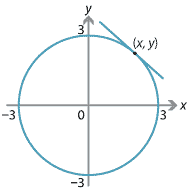## Content

### Implicit differentiation

Implicit differentiation is a powerful technique to find an instantaneous rate of change $$\dfrac{dy}{dx}$$ when there is an equation relating $$x$$ and $$y$$. It applies even when $$y$$ is not a function of $$x$$. All that is required is that there is an equation relating $$x$$ and $$y$$.

For example, consider the curve in the plane described by the equation $$x^2 + y^2 = 9$$. This is a circle centred at the origin, of radius 3.Figure : The circle $$x^2 + y^2 = 9$$.

Note that $$y$$ is not a function of $$x$$! The circle fails the vertical-line test; we have a relation, not a function. (See the module Functions I for a discussion of functions and relations.) For a given value of $$x$$, there may be two distinct values of $$y$$:

$x^2 + y^2 = 9 \quad\iff\quad y = \pm \sqrt{9 - x^2}.$

Although $$y$$ is not a function of $$x$$, we can say that the equation $$x^2 + y^2 = 9$$ expresses $$y$$ as an implicit function of $$x$$.

Suppose you want to find the gradient of the tangent to this circle at a point $$(x,y)$$. One approach would be to consider either $$f_1(x) = \sqrt{9-x^2}$$ or $$f_2(x) = - \sqrt{9-x^2}$$, depending on whether $$y$$ is positive or negative, and then differentiate. (We saw the derivative of $$\sqrt{9-x^2}$$ in exercise 17.)

The much better approach of implicit differentiation is to differentiate both sides of the equation $$x^2 + y^2 = 9$$ with respect to $$x$$. Differentiating $$y^2$$ requires the chain rule, since $$y^2$$ is a function of $$y$$ and $$y$$ is a function of $$x$$:

$\dfrac{d}{dx}(y^2) = \dfrac{d}{dy}(y^2) \, \dfrac{dy}{dx} = 2y\,\dfrac{dy}{dx}.$

We can now differentiate each term of $$x^2 + y^2 = 9$$, and we obtain

$2x + 2y\,\dfrac{dy}{dx} = 0.$

Note the power of implicit differentiation: this equation involving $$\dfrac{dy}{dx}$$ is valid for all points on the circle except $$y=0$$. When $$y \neq 0$$, we may solve for $$\dfrac{dy}{dx}$$ and obtain

$\dfrac{dy}{dx} = - \dfrac{x}{y}.$

Without implicit differentiation, we would not have found such a neat formula!

From this formula, we can see that if $$x,y$$ have the same sign, then the gradient of the tangent is negative; while if $$x,y$$ are of opposite sign, then the gradient is positive.

##### Exercise 22

Give a geometric argument why the tangent at the point $$(x,y)$$ to a circle centred at the origin has gradient $$-\dfrac{x}{y}$$.

#### Example

What is the gradient of the curve $$y^2 = x^3 - 7x$$ at the points where $$x=4$$?

#### Solution

When $$x=4$$, we have $$y^2 = 64 - 28 = 36$$, so $$y = \pm 6$$. Implicit differentiation of $$y^2 = x^3-7x$$ gives

$2y\,\dfrac{dy}{dx} = 3x^2 - 7.$

So when $$y \neq 0$$, we obtain $$\dfrac{dy}{dx} = \dfrac{3x^2-7}{2y}$$. Hence:

• At $$(x,y) = (4,6)$$, we have $$\dfrac{dy}{dx} = \dfrac{3 \cdot 4^2 - 7}{2 \cdot 6} = \dfrac{41}{12}$$.
• At $$(x,y) = (4, -6)$$, we have $$\dfrac{dy}{dx} = \dfrac{3 \cdot 4^2 - 7}{2 \cdot (-6)} = - \dfrac{41}{12}$$.

The curve in the previous example is called an elliptic curve.

##### Exercise 23

Consider the hyperbola $$x^2 - y^2 = 5$$.

1. By writing $$y$$ in terms of $$x$$, find the gradient of the tangent to the hyperbola at $$(3,-2)$$.
2. Find the same gradient by implicit differentiation.

Next page - Links forward - Higher derivatives